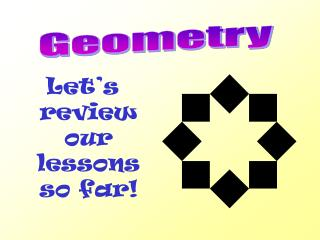DownloadDownload PresentationLet’s review our lessons so far!

# Let’s review our lessons so far!

Download Presentation## Let’s review our lessons so far!

- - - - - - - - - - - - - - - - - - - - - - - - - - - E N D - - - - - - - - - - - - - - - - - - - - - - - - - - -
##### Presentation Transcript

1. Geometry Let’s review our lessons so far!

2. Our Standards • Maryland VSC Standard • 2.A.1.b Identify and describe polygons • 2.A.1.c Identify and describe quadrilaterals • 2.B.1.a Identify and describe cubes, rectangular prisms, and triangular prisms • Maryland Technology Literacy Student Standard (MTLSS) • 3.A.1.a Use technology tools, including software and hardware, from a range of teacher-selected options to learn new content or reinforce skills

3. 15 What is a polygon? • An open figure with straight sides. • A closed figure with at least one curved side. • A closed figure with all straight sides.

4. 10 What is the name of this polygon? • hexagon • octagon • pentagon

6. 10 …and this one? • hexagon • octagon • quadrilateral

7. 10 What is the name of this polygon?... • Quadrilateral • Pentagon • Triangle

8. 10 A polygon with six sides is called a hexagon. • True • False

9. 10 A polygon with 5 vertices is called a quadrilateral. • True • False

10. 10 A three-sided polygon is called a triangle. • True • False

11. 10 An octagon always has 8 equal sides. • True • False

12. Now let’s move onto special Quadrilaterals!!! So just what is a quadrilateral? • any four sided polygon!!

13. 10 The name of this quadrilateral is… • Trapezoid • Rectangle • Rhombus

14. 10 The name of this one is? • Square • Rhombus • Trapezoid

15. 10 A square can also be called a _______. • Rhombus • Rectangle • Parallelogram • All of the above

16. 10 A quadrilateral with only one set of parallel sides is a … • Parallelogram • Trapezoid • Rectangle

17. 10 What is the name of this figure? • Cube • Rectangular Prism • Pyramid